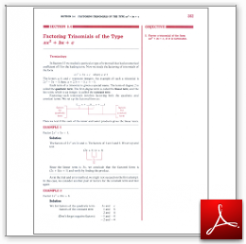Algebra Math Book - Introductory Algebra Thank you for your continual support.

 The power of mathematics rests in the logic of thinking.ID: Sec5-4
Description: Factoring Trinomials of the Type ax squared + bx + c
Price: 1.99

# Algebra Math book - Introductory Algebra - Chapter 5 - Section 4 - Factoring Trinomials of the Type ax squared + bx + c

## Section 5.4 - Objectives

5.  Factor a trinomnial of the type ax squared + bx + c, if it is factorable.

Navigate to
Previous Section or Next Section
or Chapter 5 Details or Other Chapters

This section of my algebra math book, Introductory Algebra, also includes in the download:

• Cover Sheet